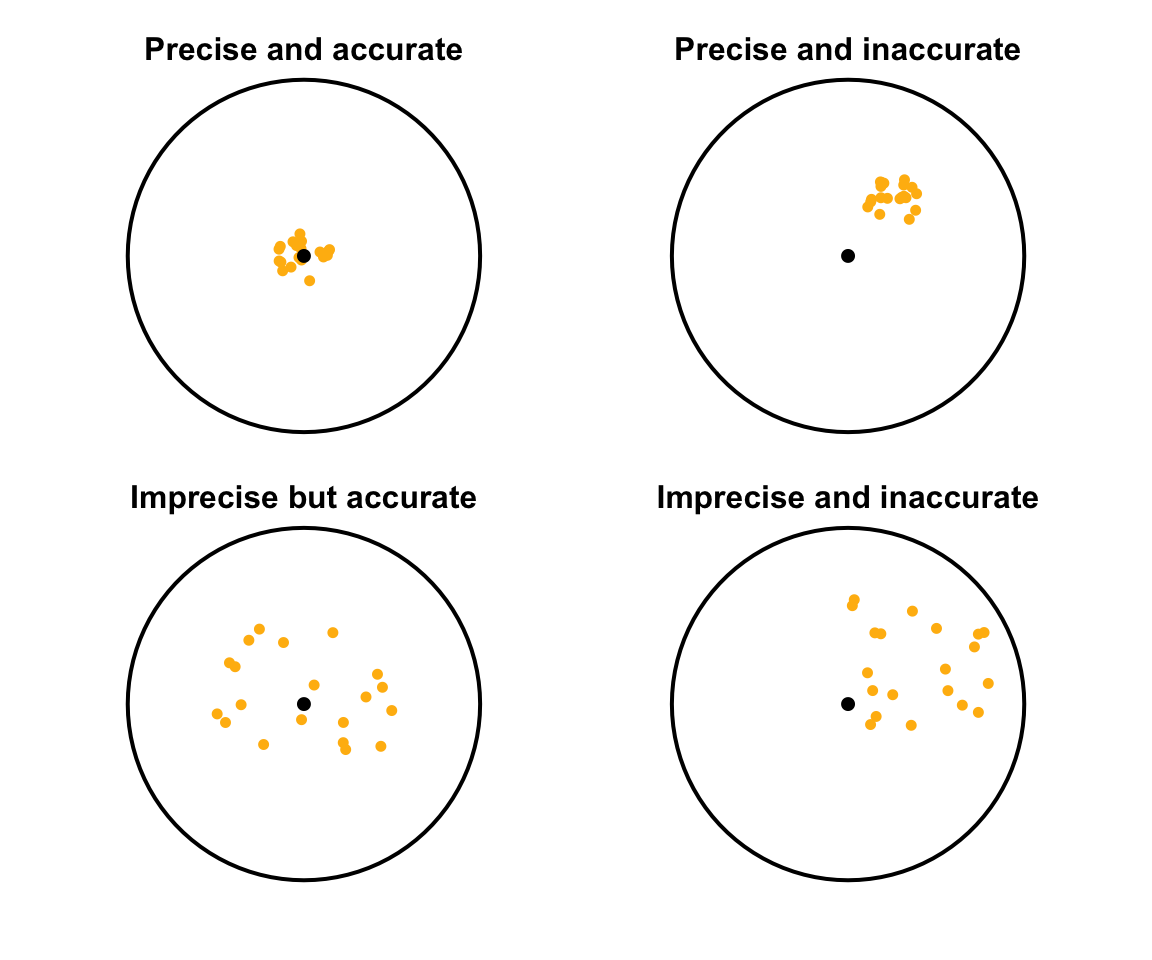## 5.2 Precision and accuracy

Two issues concerning sampling, raised in Sect. 5.1, were: which individuals should be in the sample, and how many individuals should be in the sample be. These two issues address two different aspects of sampling: precision and accuracy (Fig. 5.1).

Accuracy refers to how close a sample estimate is to the population value (on average). Precision refers to how close all the possible sample estimates are likely to be (that is, how much variation is likely in the sample estimates).

Definition 5.1 (Accuracy) Accuracy refers to how close a sample estimate is to the population value, on average.
Definition 5.2 (Precision) Precision refers to how close the sample estimates from different samples are likely to be to each other.

Using this language:

• The type of sampling (i.e., the way in which the samples in selected) impacts the accuracy of the sample estimate. In other words, the type of sampling impacts the external validity of the study.
• The size of the sample impacts the precision of the sample estimate.

For example, large samples are more likely to be precise estimates because each possible sample value will produced similar estimates, but they may or may not be accurate estimates. Similarly, random samples are likely to produce accurate estimates (and hence the study is more likely to be externally valid), but they may not be precise unless the sample is also large.FIGURE 5.1: Precision and accuracy: Each coloured dot is like a sample estimate of the population value (shown by the black central dot)Example 5.2 (Precision and accuracy) To estimate the average age of all Queenslanders, we could ask 9000 Queensland school children (a large sample indeed!).

This will give a precise answer because the sample is large, but inaccurate answer because the sample is not representative of all Queenslanders. In fact, the sample may give a precise answer to a different question: ‘What is the average age of Queensland school children?’